﻿ 类帐篷映射上的链回归点集研究 Research on Chain Regression Point Set on Tent-Like Mapping

Pure Mathematics
Vol. 09  No. 03 ( 2019 ), Article ID: 30408 , 7 pages
10.12677/PM.2019.93059

Research on Chain Regression Point Set on Tent-Like Mapping

Zhanfu Huo, Ziqing Fu

School of Mathematics and Information Science, Guangxi University, Nanning Guangxi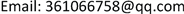Received: Apr. 29th, 2019; accepted: May 9th, 2019; published: May 24th, 2019ABSTRACT

The study of chain regression points of continuous self-mapping on metric space has always been an important part of topological dynamical system. This paper mainly studies the dynamic properties of continuous mapping on compact metric, focusing on the characteristics of chain regression points of tent-like mapping.

Keywords:Compact Metric, Continuous Mapping, Tent-Like Mapping, Chain Regression Point1. 引言与预备知识

${g}_{t,\lambda }\left(x\right)=\left\{\begin{array}{ll}\frac{1-\lambda }{t}x+\lambda \hfill & 0\le x\le t\hfill \\ \frac{x}{t-1}+\frac{1}{1-t}\hfill & t\le x\le 1\hfill \end{array}$

$t=0$ 时， ${g}_{t,\lambda }\left(x\right)=-x+1$，当 $t=1$ 时， ${g}_{t,x}\left(x\right)=\left(1-\lambda \right)x+\lambda$。记 ${a}_{t}$${g}_{t,\lambda }\left(x\right)$ 的不动点，记 ${k}_{1}$${g}_{t,\lambda }\left(x\right)$$\left[0,t\right]$ 上的斜率， ${k}_{2}=-\frac{1}{1-t}$${g}_{t,\lambda }\left(x\right)$$\left[t,1\right]$ 上的斜率。 ${U}_{\delta }^{-}=\left[0,\delta \right]$。本节主要讨论 $0 时的情形，当 $0\le \lambda \le t$ 时，记 ${a}_{t}=\frac{1}{2-t}$${{a}^{\prime }}_{t}=\frac{t+\lambda {t}^{2}-2\lambda t}{\left(1-\lambda \right)\left(2-t\right)}$，则 ${g}_{t,\lambda }\left({a}_{t}\right)={g}_{t,\lambda }\left({{a}^{\prime }}_{t}\right)={a}_{t}$，且 $0\le {{a}^{\prime }}_{t}\le t\le {a}_{t}\le 1$

2. 相关引理，因为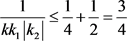，所以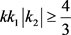，即有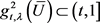，则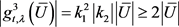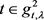，则由引理2.3，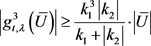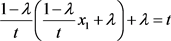，所以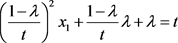，因为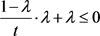，即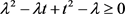，所以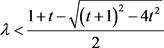时，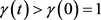，所以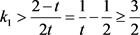。从而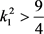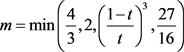，则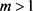，且由情形1~3知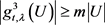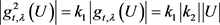.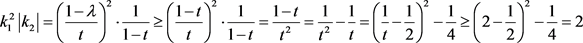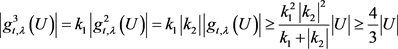.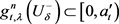；②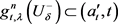；③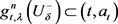；④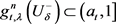； ⑤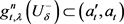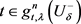3. 主要定理的证明

Research on Chain Regression Point Set on Tent-Like Mapping[J]. 理论数学, 2019, 09(03): 441-447. https://doi.org/10.12677/PM.2019.93059

1. 1. 孙太祥, 席鸿建, 张更容, 陈占和. 树映射的动为学[M]. 南宁: 广西科学技术出版社, 2011.

2. 2. 廖公夫, 王立冬, 范钦杰. 映射迭代与混純动力系统[M]. 北京: 科学出版社, 2013.

3. 3. 赵俊玲. 强链回归点及跟踪性[J]. 数学学报, 2004, 37(3): 286-291.

4. 4. 陆卫风. 帐篷映射的动力系统[J]. 苏州职业大学学报, 2004, 15(1): 65-67.

5. 5. 巧火云, 卢建平. 区间上帐篷映射的强混合性[J]. 华东交通大学学报, 2000, 17(3): 75-78.

6. 6. 熊金城. 点集拓扑讲义[M]. 北京: 高等教育出版社, 1997.

7. 7. 张景中, 熊金诚. 函数迭代与一维动力系统[M]. 成都: 四川教育出版社, 1992.

8. 8. 叶向东, 黄充, 邵松. 拓扑动力系统概论[M]. 北京: 科学出版社, 2008.